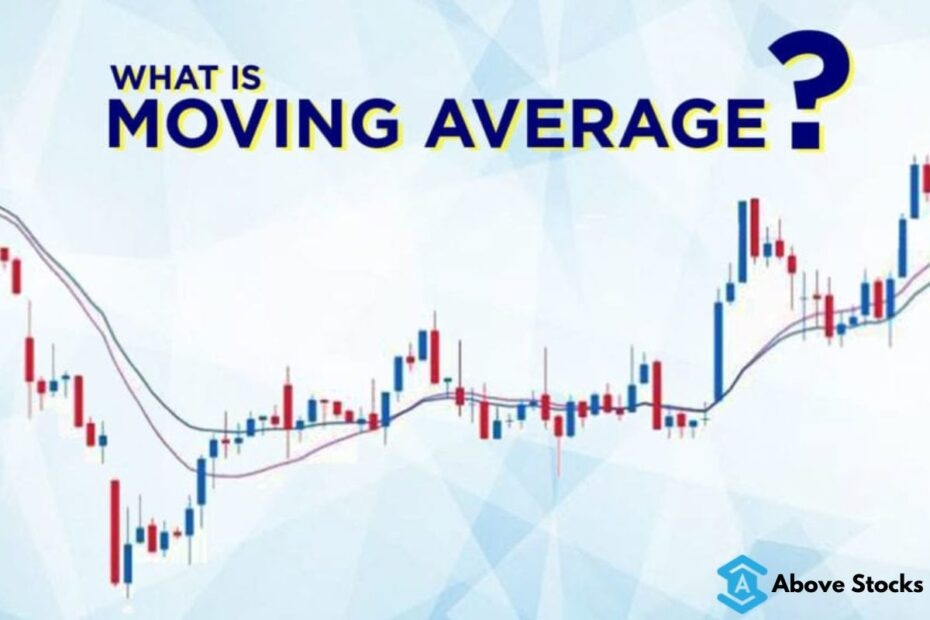# What is Moving Average? (SMA,EMA)

• LearnWe provide instant Stock Market updates on our Telegram Channel : Follow Now

Moving Averages shortly known as ‘MA’ is the most commonly used technical indicators to forecast future price movements. It is a simple technical analysis tool that smoothes out price data by creating a constantly updated average price. The average is taken over a period of time like 10, 50, 100, 200 days or hourly, 30min, 15 minor or any time frame the trader uses. The moving averages used to cut the noise on the chart. These moving averages are also used or act as support and resistance. The moving averages are used to find the trend and also to find the current trend reversal.

There are two types of moving averages Smooth Moving Average (SMA) and Exponential Moving Average (EMA). These moving averages calculated in different ways say for exp; If you are using five periods simple or smooth moving average on the one-hour chart, you would add up the closing prices for the last five hours then divide that number by five. If you plot five periods simple moving average on the 30-min chart, you would add up the closing prices of the last 150 minutes and then divide that number by five. Confused, well these all calculations are done by the charting software you just have applied it in your study.

#### Smooth Moving Average

The SMA is quite similar to a simple moving average. The SMA gives recent prices an equal weighting to historic price. The smooth moving average looks like below in the chart.

Above is the Nifty 50 hourly chart. In the above chart, 10 SMA and 20 SMA averages are applied, the blue line represents the 10SMA and yellow is 20SMA.

#### Exponential Moving Average

Another type of moving average is the Exponential Moving Average (EMA). It applies more weighting to the most recent price. It is also the same as Smooth Moving Average but if you plot a 50-day SMA and 50-day EMA on the same chart, you will notice that the EMA reacts more quickly to price changes than the SMA because it applies more weight to the most recent price.

From the chart above it can be seen that the 50-day SMA and 50-day EMA applied on the same chart. It can be seen that the 50-day EMA pink rises quicker and also begins to fall quicker than the 50-day SMA.

One type of moving average can’t be better than another. An EMA can work better on a stock for a time, and at another time SMA may work better for the same stock.

Now the more important is that the time frame chosen for a moving average also playing an important role in its effectiveness regardless of its type.

More importantly how to use and trades Moving averages well,

From the above first chart, if you see and examine then you will find that the price is staying above and moving up once the moving averages crossed below the price of the index.

In the second chart, if you see then you will find that the price tends to up once the moving averages crossed the price below and then it comes down once the moving average crossed up.

The SMA is a consider as lagging indicator because it gives signal slowly, but the EMA is giving a fast signal as it has more weight.